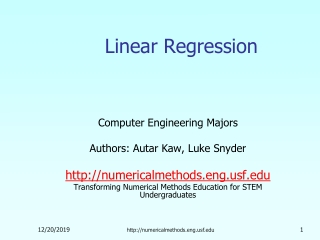Download PresentationLinear Regression

Linear Regression

Télécharger la présentationLinear Regression

- - - - - - - - - - - - - - - - - - - - - - - - - - - E N D - - - - - - - - - - - - - - - - - - - - - - - - - - -
Presentation Transcript

1. Linear Regression Computer Engineering Majors Authors: Autar Kaw, Luke Snyder http://numericalmethods.eng.usf.edu Transforming Numerical Methods Education for STEM Undergraduates http://numericalmethods.eng.usf.edu

2. Linear Regressionhttp://numericalmethods.eng.usf.edu

3. What is Regression? What is regression?Given n data points best fit to the data. The best fit is generally based on minimizing the sum of the square of the residuals, . Residual at a point is Sum of the square of the residuals Figure. Basic model for regression http://numericalmethods.eng.usf.edu

4. y x Linear Regression-Criterion#1 Given n data points best fit to the data. Figure. Linear regression of y vs. x data showing residuals at a typical point, xi . Does minimizing work as a criterion, where http://numericalmethods.eng.usf.edu

5. Example for Criterion#1 Example: Given the data points (2,4), (3,6), (2,6) and (3,8), best fit the data to a straight line using Criterion#1 Table. Data Points Figure. Data points for y vs. x data. http://numericalmethods.eng.usf.edu

6. Linear Regression-Criteria#1 Using y=4x-4 as the regression curve Table. Residuals at each point for regression model y = 4x – 4. Figure. Regression curve for y=4x-4, y vs. x data http://numericalmethods.eng.usf.edu

7. Linear Regression-Criteria#1 Using y=6 as a regression curve Table. Residuals at each point for y=6 Figure. Regression curve for y=6, y vs. x data http://numericalmethods.eng.usf.edu

8. Linear Regression – Criterion #1 for both regression models of y=4x-4 and y=6. The sum of the residuals is as small as possible, that is zero, but the regression model is not unique. Hence the above criterion of minimizing the sum of the residuals is a bad criterion. http://numericalmethods.eng.usf.edu

9. y x Linear Regression-Criterion#2 Will minimizing work any better? Figure. Linear regression of y vs. x data showing residuals at a typical point, xi . http://numericalmethods.eng.usf.edu

10. Linear Regression-Criteria 2 Using y=4x-4 as the regression curve Table. The absolute residuals employing the y=4x-4 regression model Figure. Regression curve for y=4x-4, y vs. x data http://numericalmethods.eng.usf.edu

11. Linear Regression-Criteria#2 Using y=6 as a regression curve Table. Absolute residuals employing the y=6 model Figure. Regression curve for y=6, y vs. x data http://numericalmethods.eng.usf.edu

12. Linear Regression-Criterion#2 for both regression models of y=4x-4 and y=6. The sum of the errors has been made as small as possible, that is 4, but the regression model is not unique. Hence the above criterion of minimizing the sum of the absolute value of the residuals is also a bad criterion. Can you find a regression line for which and has unique regression coefficients? http://numericalmethods.eng.usf.edu

13. y x Least Squares Criterion The least squares criterion minimizes the sum of the square of the residuals in the model, and also produces a unique line. Figure. Linear regression of y vs. x data showing residuals at a typical point, xi . http://numericalmethods.eng.usf.edu

14. Finding Constants of Linear Model Minimize the sum of the square of the residuals: To find and we minimize with respect to and . giving http://numericalmethods.eng.usf.edu

15. Finding Constants of Linear Model Solving for and directly yields, and http://numericalmethods.eng.usf.edu

16. Example 1 To simplify a model for a diode, it is approximated by a forward bias model consisting of DC voltage, and resistor . Below are the current vs. voltage data that is collected for a small signal. Table. Data points for I vs. V Figure. Data points for I vs. V data. http://numericalmethods.eng.usf.edu

17. Example 1 cont. The I vs. V data is regressed to Once and are known, and can be calculated as and Find the value of and . http://numericalmethods.eng.usf.edu

18. Example 1 cont. The necessary summations are given as, With Table. Necessary summations for the calculation of constants for linear model. http://numericalmethods.eng.usf.edu

19. Example 1 cont. We can now calculate using where http://numericalmethods.eng.usf.edu

20. Example 1 cont. This gives the equation as our linear regression model. Figure. Linear regression of current vs. voltage http://numericalmethods.eng.usf.edu

21. Example 2 To find the longitudinal modulus of composite, the following data is collected. Find the longitudinal modulus, using the regression model and the sum of the square of the Table. Stress vs. Strain data residuals. Figure. Data points for Stress vs. Strain data http://numericalmethods.eng.usf.edu

22. Example 2 cont. Residual at each point is given by The sum of the square of the residuals then is Differentiate with respect to Therefore http://numericalmethods.eng.usf.edu

23. Example 2 cont. Table. Summation data for regression model With and Using http://numericalmethods.eng.usf.edu

24. Example 2 Results The equation describes the data. Figure. Linear regression for Stress vs. Strain data http://numericalmethods.eng.usf.edu

25. Additional Resources For all resources on this topic such as digital audiovisual lectures, primers, textbook chapters, multiple-choice tests, worksheets in MATLAB, MATHEMATICA, MathCad and MAPLE, blogs, related physical problems, please visit http://numericalmethods.eng.usf.edu/topics/linear_regression.html

26. THE END http://numericalmethods.eng.usf.edu